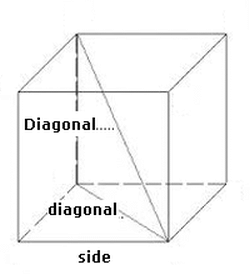### Cube

A cube is a three-dimensional solid figure to six identical square faces.
Elements of cube:
• Face: parallelogram with four equal sides.
• Base: horizontal face.
• Total surface: sum of the six face areas.
• Lateral surface: sum of the four face areas.
• Space diagonal: the segments line, joining two opposite vertices, not belonging to the same face. Also called cube diagonal
• Face diagonal: the segments line, joining two opposite corners of the same face.
Enter data using the point as a decimal separator. Ex 3,750.17 enter: 3750.17; The results will be shown after a click on Calculate.Cube Decimal places: Side           Volume Space diagonal Radius inscribed sphera Radius circunscribed sphera Total surface: Lateral surface: Area of the bases: Volume: Face diagonal: Space diagonal: Radius inscribed sphera: Radius circunscribed sphere: Volume inscribed sphera: Volume circunscribed sphere: Note:

Note:The accuracy of the calculator and its applicability to particular cases is not guaranteed.